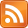A great example of Ruby on Rails "magic" is this:

2.5.1 :001 > 1.day.ago
=> Wed, 06 May 2020 20:23:24 UTC +00:00


Very convenient. When I was a beginner to Rails, I thought about 1.day.ago as an irreducible incantation. But if you think about what is happening beneath the hood, it's a bit goofy. The numeral 1 has a method .day, which returns an object which has a method .ago, which returns the date — that is, an object of type ActiveSupport::TimeWithZone.

Thinking about it this way suggests a question: what is that intermediate object returned by .day?

2.5.1 :001 > 1.day
=> 1 day
2.5.1 :002 > 1.day.class
=> ActiveSupport::Duration


There are two kinds of time objects: ActiveSupport::TimeWithZone for points in time (datetimes), and ActiveSupport::Duration for distances between points in time. These are different concepts! And you need different ones at different times. You can't add points in time to each other; what's last Friday plus last Sunday? You can only add durations to points in time: last Friday plus one week.

I remember screwing up this distinction several times when I was a beginning programmer. I'm pretty sure I did things like use python's datetime class with zeroes in everything but the minutes slot to represent a duration. It's nice that the type system stops us here.

2.5.1 :001 > 1.day.ago + 1.day
=> Thu, 07 May 2020 20:38:37 UTC +00:00
2.5.1 :002 > 1.day + 1.day
=> 2 days
2.5.1 :003 > 1.day.ago + 1.day.ago
Traceback (most recent call last):
1: from (irb):1
TypeError (not an integer)


Now here's a new set of goggles to look at this through. In math, an affine space is a set like this where we have points and vectors between them, and we can take vector + point or vector + vector, but not point + point.

Alternatively, you can see an affine space as a vector space, but if we don't care where the origin is. To see this in the time example, consider that a datetime object can be implemented by storing an integer representing the number of milliseconds since midnight on 1 January 1970 UTC, the "Unix epoch."

This is an arbitrary point in time which functions as our "zero." If you wanted, you could write a sum_two_datetimes(a, b) routine which simply added a.milliseconds to b.milliseconds. But the result would be nonsense. We can call it nonsense in a principled way by noting that it would depend on the choice of zero. The difference of two datetimes is the same regardless of whether the Unix epoch is in 1970 or 1971, but not the sum. Since we do not want calculations on datetimes to depend on the choice of zero, we must forbid this operation.

Hence, an affine space is a vector space where we don't want our calculations to depend on where the origin is.

Another good example of an affine space is... space. Like the 3D space we live in, you know? (Mathematicians call this $\mathbb{R}^3$.) "But wait," you say, "$\mathbb{R}^3$ is a vector space, not an affine space!" Well, what's $\text{Paris} + \text{London}$ then? The result depends on your choice of zero (if you choose the center of the Earth as zero, the location represented by the sum is out in space somewhere, while if you choose Paris as zero, the location represented by the sum is London). Meanwhile, $\text{Paris} - \text{London}$ is always the same thing; the vector pointing from London to Paris.

I learned about $\mathbb{R}^3$ in a multivariable calculus with a very exciting teacher and it was very exciting. We were going to map out the physical world! We put a point $P$ on the board and it was just a point in the world! "What is a vector?" we were asked, and we chanted "It's just a point!" Then there was a distinction made between "vectors," which were locations in space, and "free vectors," which were just kind of floating around.

If you treat points in 3D space as elements of $\mathbb{R}^3$, it's weird because you better not add them, even though the rules let you. For this reason, I think the 3D space we live in is best modeled as an affine space, where locations are the points and the vectors are in $\mathbb{R}^3$. I'm sympathetic to not having to introduce the abstract definition of an affine space in your multivariable class, but I think it would have improved my understanding of the theorems if we noted explicitly which things are locations and which are vectors between locations. And it doesn't seem so much more complicated.